Solve system of linear equations by inverse matrix method online

The one famous method of solving systems of linear algebraic equations (SLAE) is the inverse matrix method. Suppose we have SLAE of two equations with two unknowns.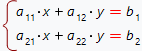Intoduce the notations: A - SLAE's matrix of the form: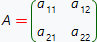X - column vector of unknowns which are to be found: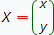B - vector column of free coefficients: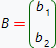So, the initial SLAE can be rewritten in matrix notations: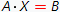In order to solve this matrix equation, multiply both its sides from the left by A-1 matrix: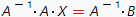Here, A-1 - is inverse matrix of matrix A. Such matrix exists for any square nondegenerate matrix (i.e. its determinant doesn't equal to zero).

The conditions above show the boundaries of application of inverse matrix method for SLAE solution. First of all: SLAE's matrix A must be square. This means, that the number of equations must be equal to the number of variables. In the second place: the determinant of matrix A must not be equal to zero: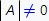In addition, the inverse matrix shares wonderful feature: its product with initial matrix is commutative and equals to the identity matrix: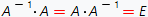Returning to the solution of our matrix equation, we get: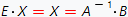So, in order to solve SLAE by inverse matrix method, first of all one need to check, that inverse matrix does exist and then find it and multiply by the vector B.

The purpose of our online calculator is to solve SLAE by inverse matrix method. The calculator finds the step by step solution. The SLAE's equations are entered in their natural form. The equation's coefficient might be not only number and fractions, but also the parameters - in this case the calculator gives solution is common form.

SLAE solve by inverse matrix method
,,
allow automatic SLE variables detection.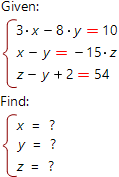System of linear algebraic equations you want to solve: